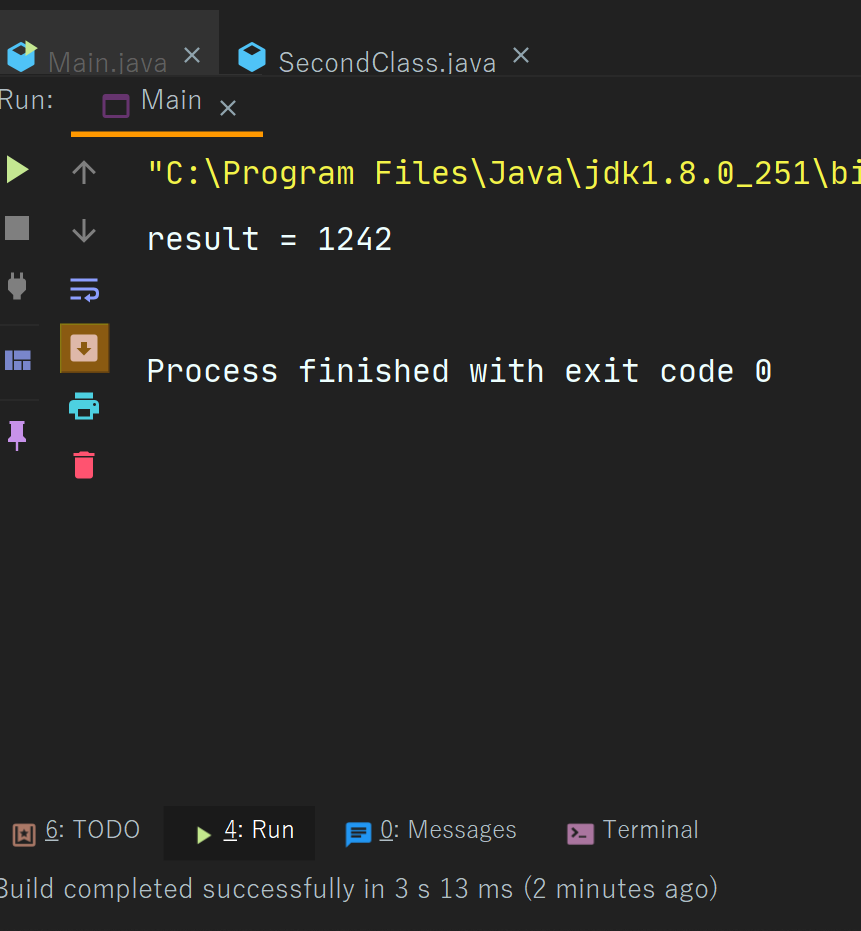Java Multiplication Operators

# Java Multiplication Operators

Multiplication Operator In Java
The multiplication operator in java is represented by the star sign which is indicated as  - “ * ”.
The multiplication operator is used to multiply a value from another value.
It is also a part of the binary arithmetic operator which means that this operator is used on two operators.
Now we will see Example -
Example  -
 //online promotion house //web designing house   import second.*; import java.util.*;     class Main{   public static void main(String[] args) {       int a = 54;       int b = 23;       int result  = a*b;                 System.out.println("result = "+result1);         }   }
Output -